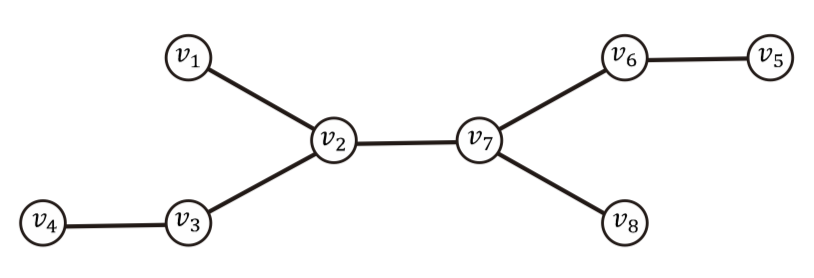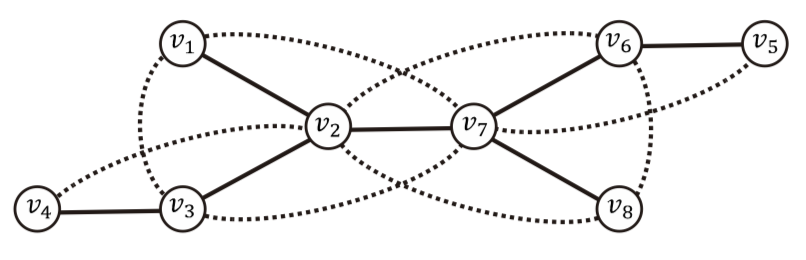시간 제한메모리 제한제출정답맞힌 사람정답 비율
3 초 512 MB114241616.327%

## 문제

The square T2 of a tree T is defined as a simple undirected graph with the same vertex set as T and the edge set that is augmented in such a way that two vertices of T2 are adjacent if and only if there exists a path of length at most two in T joining them. That is, its vertex set is equal to that of T and its edge set is equal to {(u, v) : dT(u, v) ≤ 2}, where dT(u, v) denotes the distance between u and v in T. Figure I.1 shows a tree and its square.(a) a tree T (b) its square T2

Figure I.1: A tree T and its square T2. An edge of T2 that joins vertices u and v with dT(u, v) = 2 is shown by a dotted curve.

If a graph G is the square of some tree T, i.e. G = T2, then T is said to be a square root of G. For a given tree T, computing the square T2 is trivial; for a given graph G, however, deciding if there exists a tree T such that T2 = G is not trivial. Your job is to write an efficient running program for deciding whether or not there exists a tree that is a square root of an input graph G.

## 입력

Your program is to read from standard input. The first line contains two positive integers n and m, respectively, representing the numbers of vertices and edges of the input graph G, where 2 ≤ n ≤ 100,000 and m ≤ 1,000,000. It is followed by m lines, each contains two positive integers u and v representing an edge between the vertices u and v of G. It is assumed that G is a simple undirected graph whose vertices are indexed from 1 to n.

## 출력

Your program is to write to standard output. The first line must contain an integer indicating whether there exists a tree that is a square root of the input graph. If yes, the integer must be 1; otherwise -1. When and only when the first line is 1, it must be followed by the description of an arbitrary tree that is a square root of the input graph. A tree is described by a single line containing an integer n, representing the number of vertices, followed by n − 1 lines, each contains two positive integers u and v representing an edge between the vertices u and v of the tree.

## 예제 입력 1

8 15
1 2
1 3
1 7
2 3
2 4
2 6
2 7
2 8
3 4
3 7
5 6
5 7
6 7
6 8
7 8


## 예제 출력 1

1
8
1 2
2 3
3 4
7 2
5 6
6 7
7 8


## 예제 입력 2

8 14
1 2
1 3
1 7
2 3
2 4
2 6
2 7
2 8
3 4
3 7
5 6
5 7
6 7
6 8


## 예제 출력 2

-1


## 예제 입력 3

5 7
1 2
2 3
3 4
4 5
5 3
4 2
3 1


## 예제 출력 3

1
5
1 2
2 3
3 4
4 5


## 예제 입력 4

4 6
1 2
2 3
3 4
4 1
4 2
3 1


## 예제 출력 4

1
4
2 1
3 1
4 1